Courses

# Test: Transformer of Electrical Machines

## 10 Questions MCQ Test Topicwise Question Bank for Electrical Engineering | Test: Transformer of Electrical Machines

Description
This mock test of Test: Transformer of Electrical Machines for Electrical Engineering (EE) helps you for every Electrical Engineering (EE) entrance exam. This contains 10 Multiple Choice Questions for Electrical Engineering (EE) Test: Transformer of Electrical Machines (mcq) to study with solutions a complete question bank. The solved questions answers in this Test: Transformer of Electrical Machines quiz give you a good mix of easy questions and tough questions. Electrical Engineering (EE) students definitely take this Test: Transformer of Electrical Machines exercise for a better result in the exam. You can find other Test: Transformer of Electrical Machines extra questions, long questions & short questions for Electrical Engineering (EE) on EduRev as well by searching above.
QUESTION: 1

### Which of the following is not true relating to an ideal transformer?

Solution:

In an ideal transformer there is no-loss (either in winding or core). The leakage reactance is also zero (as no-voltage drop).
Magnetizing current is zero due to infinite permeability of core.

QUESTION: 2

### The emf per turn of a single phase 10 kVA, 2200/220 V, 50 Hz transformer is 10 V. The net cross-sectional area of core for a maximum flux density of 1.5 T is

Solution:

Maximum flux,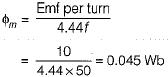∴ New cross-sectional area of core,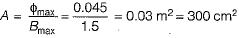QUESTION: 3

### A 1 kVA, 400 Hz transformer is desired to be used at a frequency of 60 Hz. The kVA rating of the transformer at this reduced frequency is

Solution:

kVA rating of transformer,
or,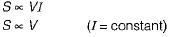Since V ∝ f, therefore, S ∝ f
∴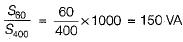QUESTION: 4

A 2200 / 250 V transformer takes 0.5 A at a p.f. of 0.3 on open-circuit. The magnetising component of no-load primary current is

Solution: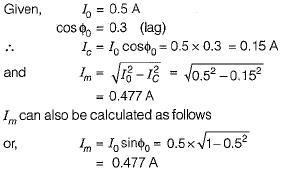QUESTION: 5

A 50 MVA, 76.2/33 kV, 1-φ, 50 Hz, two-winding transformer with tap changer has percentage impedance of (0.5 + j7.0). What tapping must be used to maintain rated voltage at the secondary on full load at 0.8 lagging power factor?

Solution: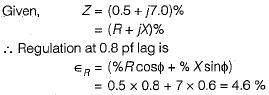Hence, voltage to be raised, for maintaining rated voltage = 4.6%.
Thus, tap setting required on the HV side = -4.6% or 4.6 % down.

QUESTION: 6

The ohmic drop of a transformer is 1% while the reactance drop is 5% of the voltage. The regulation of the transformer at 0.8 power factor leading and 0.8 power factor lagging are respectively

Solution: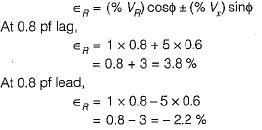QUESTION: 7

A 1 kV, 50 Hz supply to a transformer results in 650 W hysteresis loss and 400 W eddy current loss. If both the applied voltage and frequency are doubled, the new core loss would be

Solution: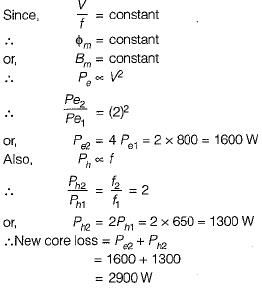QUESTION: 8

A transformer designed for operation of 60 Hz supply is working on 50 Hz supply system without changing its voltage and current ratings. When compared with full-load efficiency at 60 Hz, the transformer efficiency on full load at 50 Hz will

Solution:

We know that, eddy current loss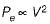and hysteresis loss,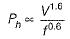When V - constant, Pe = constant
and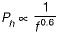When frequency is increased from 50 Hz to 60 Hz, Ph will increase. Thus, core loss will increase marginally, therefore efficiency will decrease marginally.

QUESTION: 9

For a core-type power transformers, both primary and secondary windings have circular coil sections, because this section

Solution:

Primary and secondary windings of a core type transformer have circular coil section to reduce the mean length of each turn so that it require minimum conductor material and thus have less copper loss which will increase the efficiency.

QUESTION: 10

A 10 kVA, 400 V/ 200 V, 1 -phase transformer with a percentage resistance of 3% and percentage reactance of 6% is supplying a current of 50 A to a resistive load. The value of the load voltage is

Solution: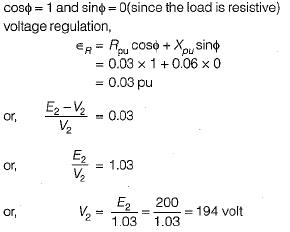Hence, load voltage = 194 volt